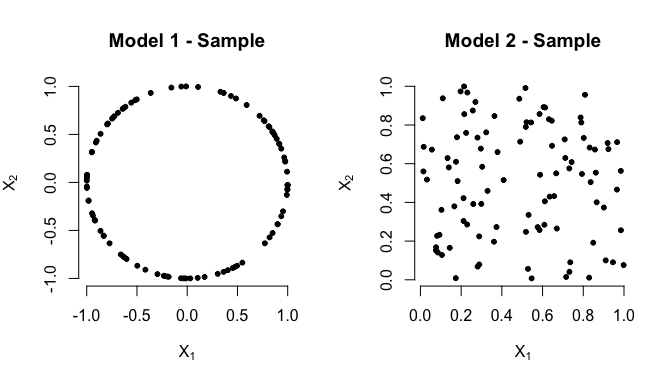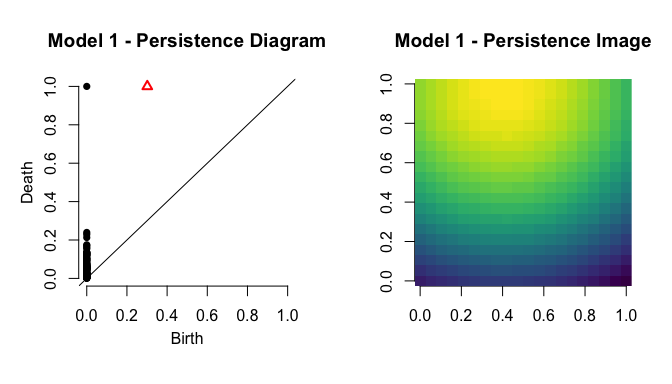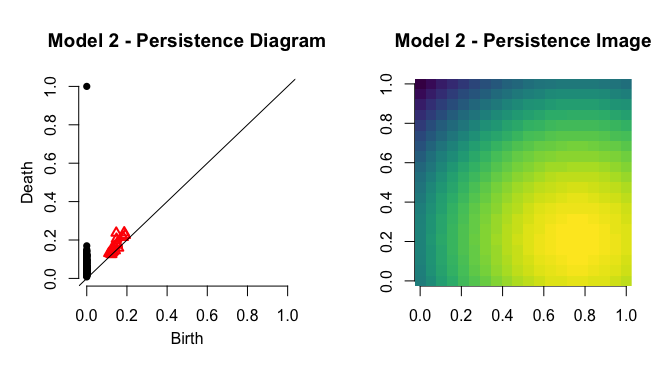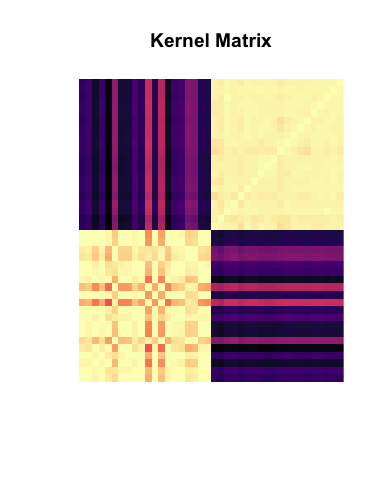kernelTDA - Vignette

Statistical Learning with Kernels for Topological Data Analysis

Topological Data Analysis (TDA) is a relatively new branch of statistics devoted to the estimation of the connectivity structure of data, via means of topological invariants such as (Persistent) Homology Groups. Topology provides a highly interpretable characterization of data, making TDA ever more popular in a time when data are becoming the era of big and complex hence TDA has seen an impressive growth in the last couple of years.

While several inference-ready tools have been developed in the framework of TDA, they are still o The hype on the theoretical side however, has not been matched by the same popularity in applications. The kernelTDA package aims at filling this gap by providing — tools, with a special emphasis on kernels. The main contribution of kernelTDA is in fact to provide an R implementation of the most popular kernels to be used in the space of Persistence Diagrams:

In addition, it also contains an R interface to the C++ library HERA, which allows to compute any Wasserstein distance between Persistence Diagrams.

Preliminaries - some definitions

Before showing how to use the functions contained in this package, we briefly recap how those that we are going to use in this vignette are defined.

Given two Persistence Diagrams $$D_1$$, $$D_2$$, we define the

• $$L_p$$ $$q-$$Wasserstein distance: $W_{p,q} (D_1, D_2) = \left[ \inf_{\gamma} \sum _{x\in D_1 } \parallel x - \gamma (x)\parallel_p^q \right]^{\frac{1}{q}}$ where the infimum is taken over all bijections $$\gamma : D_1 \mapsto D_2$$, and $$\parallel \cdot\parallel_p$$ is the $$L_p$$ norm.

• Persistence Scale Space Kernel: $K_{\text{PSS}}(D_1, D_2) = \frac{1}{8\pi\sigma}\sum_{x \in D_1} \sum_{y \in D_2} \mathtt{e}^{-\frac{\parallel x-y \parallel ^2}{8\sigma}} - \mathtt{e}^{-\frac{\parallel x-\bar{y} \parallel^2}{8\sigma}}$

• Geodesic Wasserstein Kernel(s)

• Gaussian: $K_{\text{GG}}(D_1, D_2) = \exp\left\{\frac{1}{h} W_{2,2}(D_1, D_2)^2 \right\}$

• Laplacian: $K_{\text{GL}}(D_1, D_2) = \exp\left\{\frac{1}{h}W_{2,2}(D_1, D_2) \right\}$

The package - some toy examples

Let us consider two generating models for our data:

• Model 1: a uniform distribution on the unit radius circle;

• Model 2: a uniform distribution on the unit square [0,1] x [0,1].

The following code produces two samples of $$n=100$$ observations from the different models.

library(TDA)
x1 = circleUnif(100)
x2 = cbind(runif(100), runif(100))

Using the package TDA we can build a Persistence Diagram from each sample, as follows:

diag1 = ripsDiag(x1, maxdimension = 1, maxscale = 1)$diagram diag2 = ripsDiag(x2, maxdimension = 1, maxscale = 1)$diagram

Figure shows us the two sample and their corresponding Persistence Diagrams.The first intuitive way to compare the two objects is by graphical inspection. In addition to their Persistence Diagrams, we can also compare their Persistence Images, which we implement in this package. As by definition these two samples have a very different structure in terms of cycles (there is $$1$$ cycle in Model $$1$$, while there should be none in Model $$2$$), we set dimension = 1, in order to focus on topological features of dimension $$1$$.

library(kernelTDA)
pi1 = pers.image(diag1, nbins = 20, dimension = 1, h = 1)
pi2 = pers.image(diag2, nbins = 20, dimension = 1, h = 1)

This results in the following:

#> Registered S3 methods overwritten by 'ggplot2':
#>   method         from
#>   [.quosures     rlang
#>   c.quosures     rlang
#>   print.quosures rlangA more formal way to compare the two is through a Wasserstein distance. Let us consider for example the Geodesic distance on the space of Persistence Diagrams, i.e. the $$L_2$$ $$q$$-Wasserstein distance, and let us take $$q = 1$$:

wasserstein.distance(d1 = diag1, d2 = diag2, dimension = 1, q = 1, p = 2)
#>  0.8225564

Learning with topology

Assume now our data come already in the form of persistence diagrams, and that we have $$20$$ of them from each of the two different models; the kernels provided in this package allow to use any ready made kernel algorithm to perform standard statistical analysis on these unusual data.

Let us consider for example clustering. Suppose we have stored the diagrams in a list called foo.data, whose first $$20$$ elements are diagram from Model $$1$$ and last $$20$$ from Model $$2$$. We can build a kernel matrix as:and then feed it into any standard kernel algorithm. If we choose to use kernel spectral clustering as implemented in the package kernlab for example:

As we could expect, the cluster labels recover perfectly the structure we gave to our dataset:

Analogously, if we want to classify the diagrams we can use a kernel Support Vector algorithm such as:

The Geodesic Gaussian and the Geodesic Laplacian Kernels are however not positive semi-definite, hence the standard SVM solver cannot be directly used with them. To overcome this problem we implemented the extension of kernel Support Vector Machine to the case of indefinite kernels introduced by Loosli et al.. The implementation is largely based on the C++ library LIBSVM, and on its R interface in the package e1071.

In order to perform the same classification task as before using the already computed Geodesic Gaussian kernel we can use the following function:

returning a perfect classification (at least in this trivial example). Notice that as the Krein SVM solver is a generalization of the standard SVM solver, when fed with a positive semidefinite kernel matrix, the results of the two methods will be the same, hence the function krein.svm can be used with the other kernels of this packages without performance loss.